This question is inspired from Example 13 - Chapter 15 Class 10 - Probability

## Rahul and Ravi planned to play Business ( board game) in which they were supposed to use two dice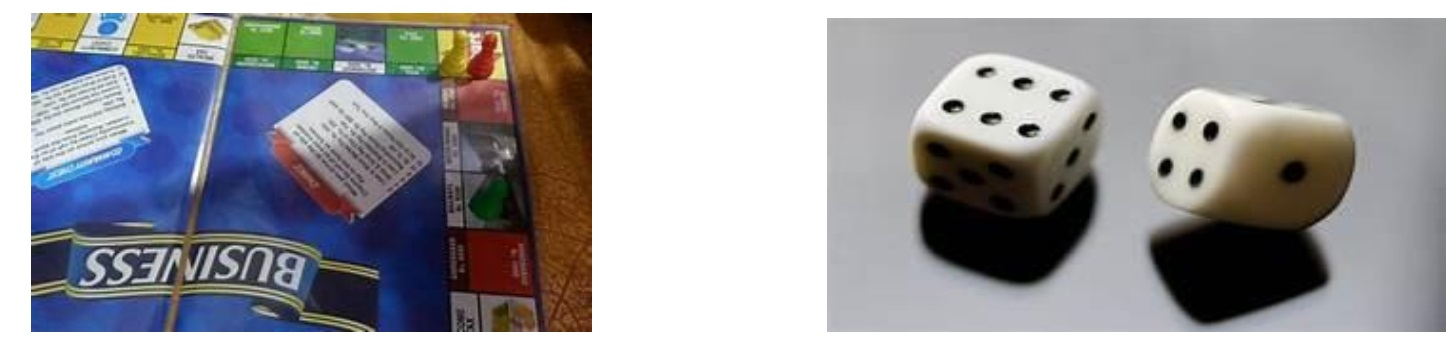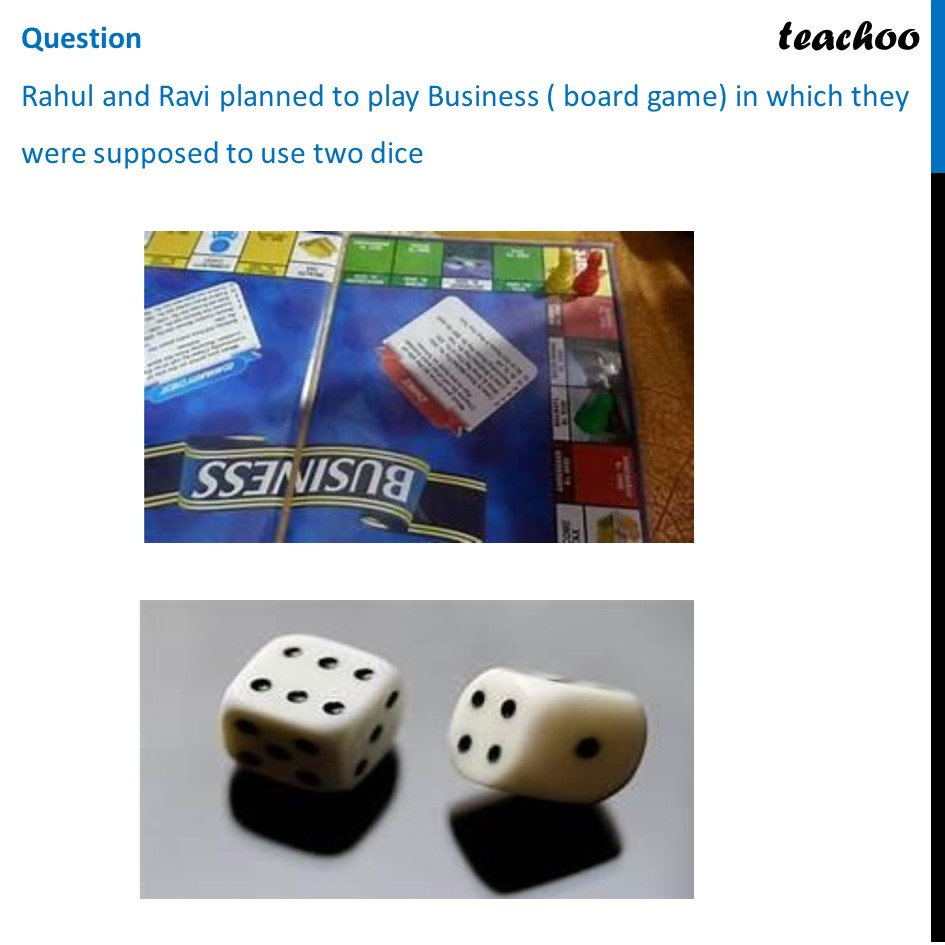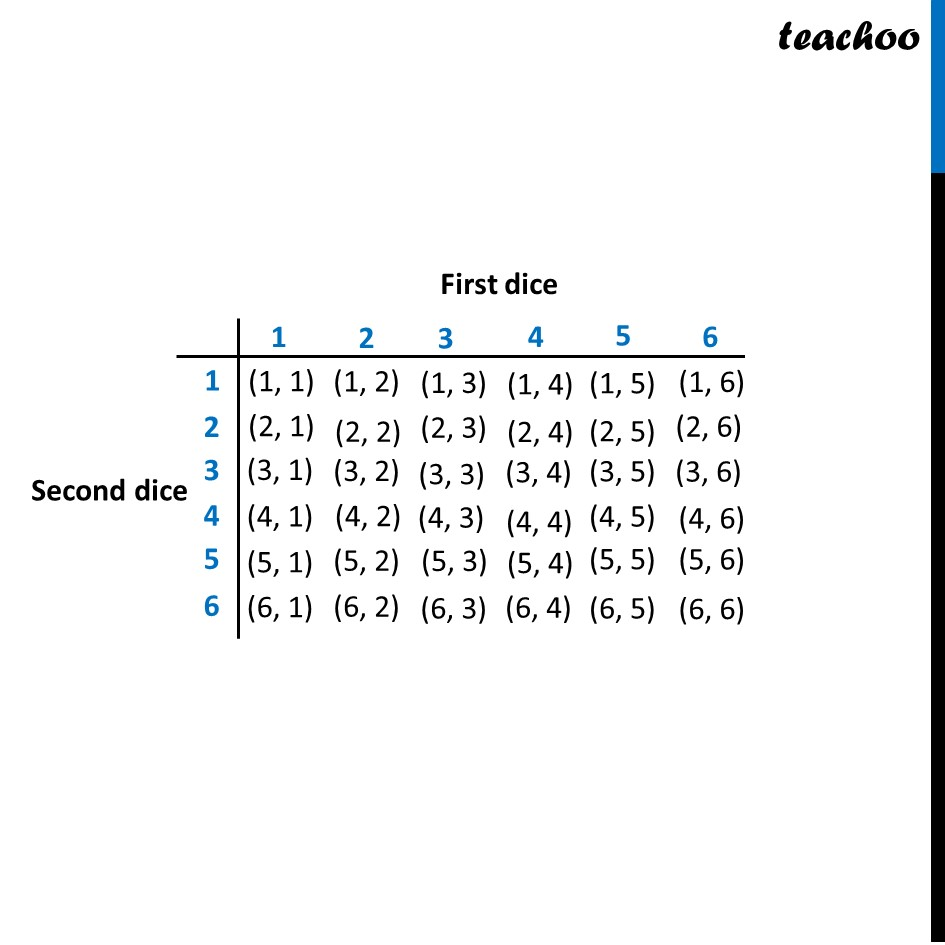## (a) 1/26                 (b) 5/36                 (c) 1/18                     (d) 0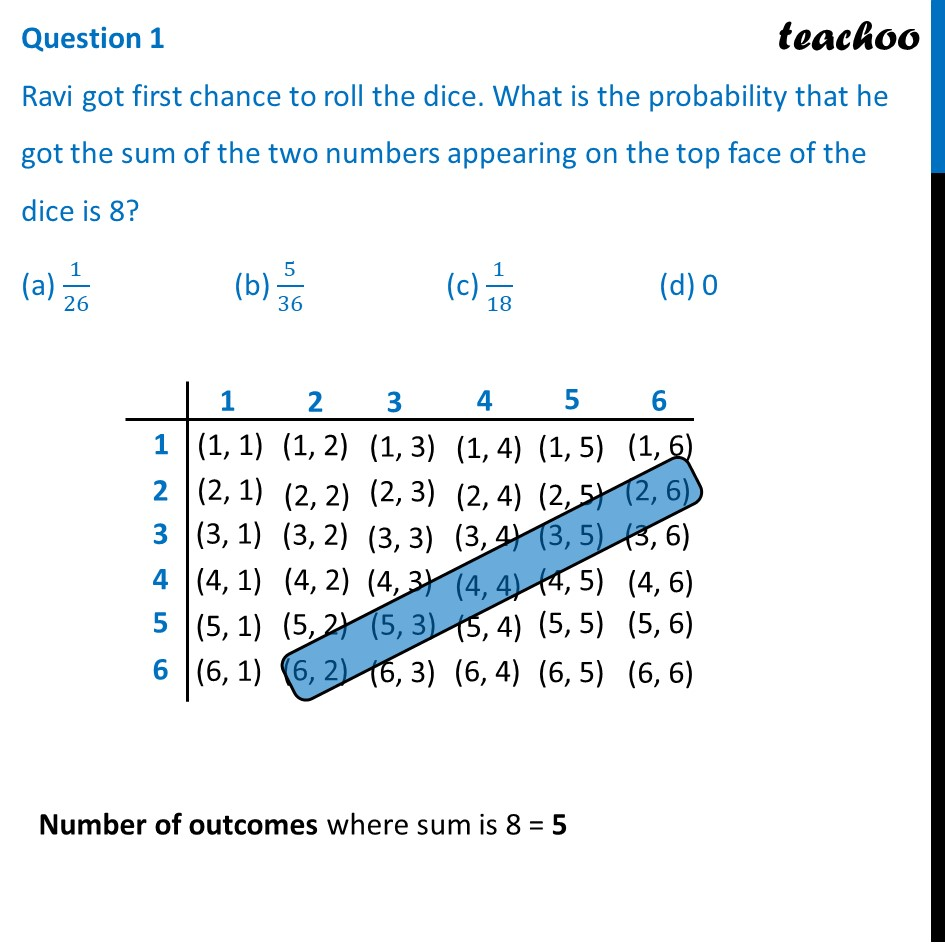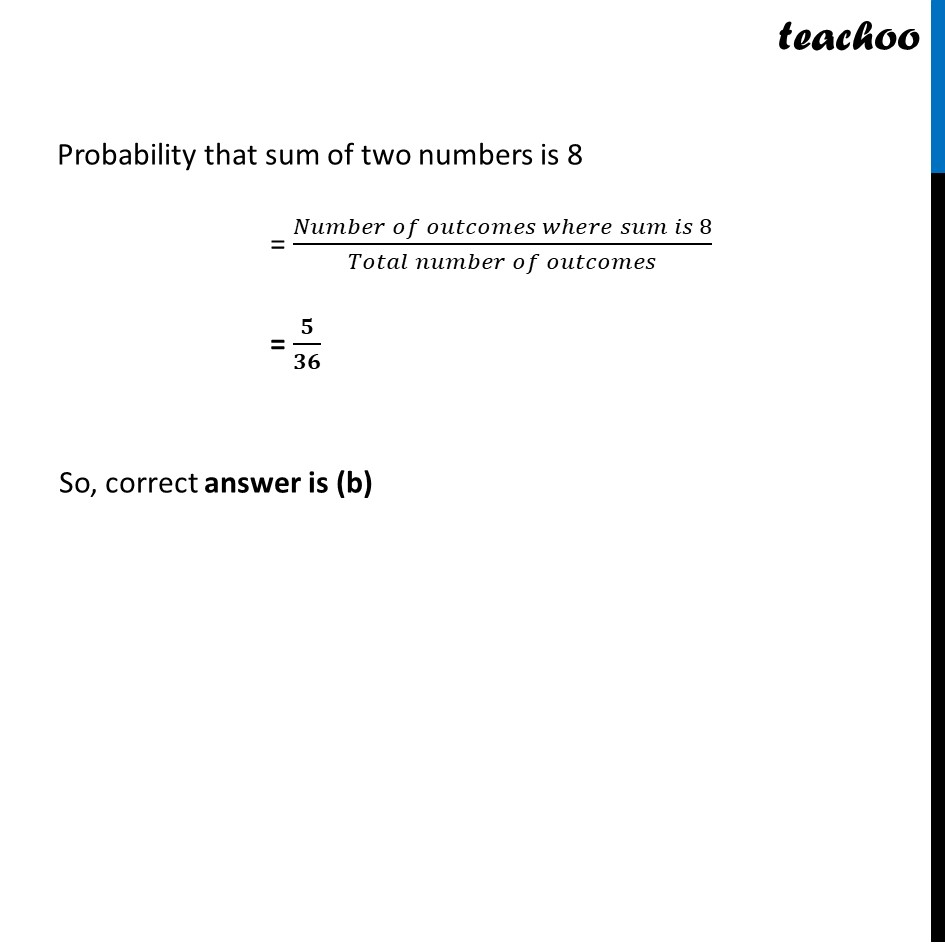## (a) 1                     (b) 5/36                    (c) 1/18                     (d) 0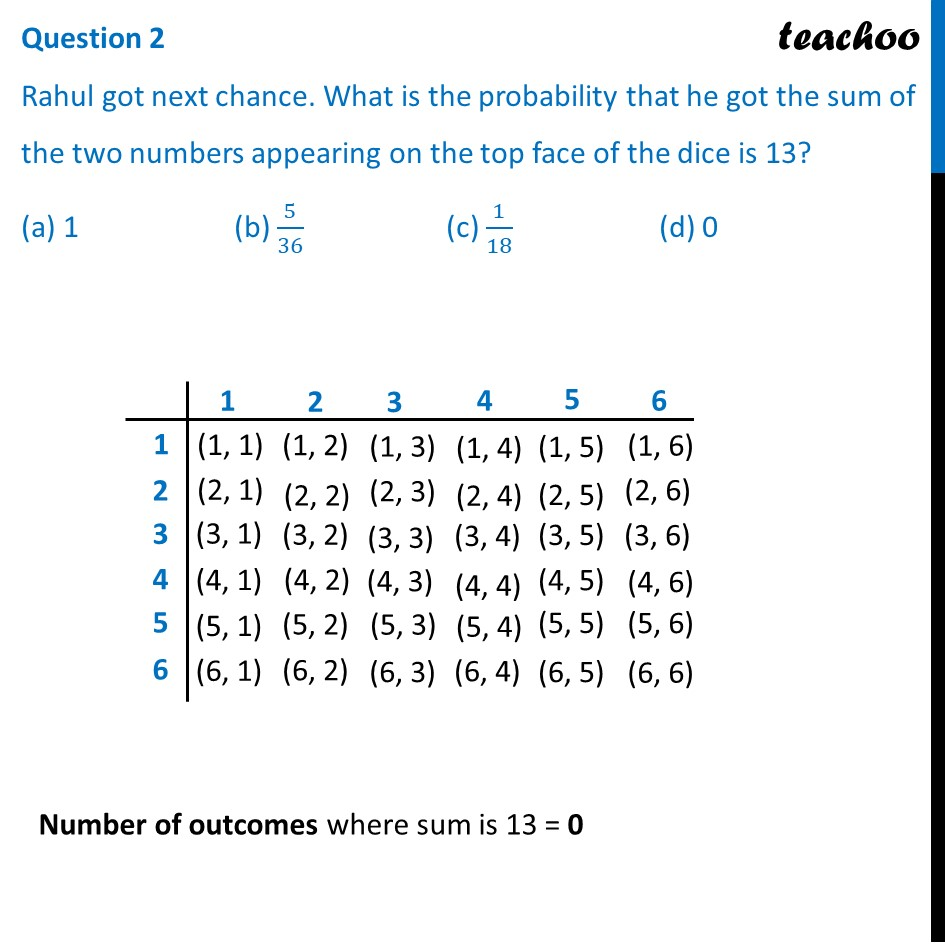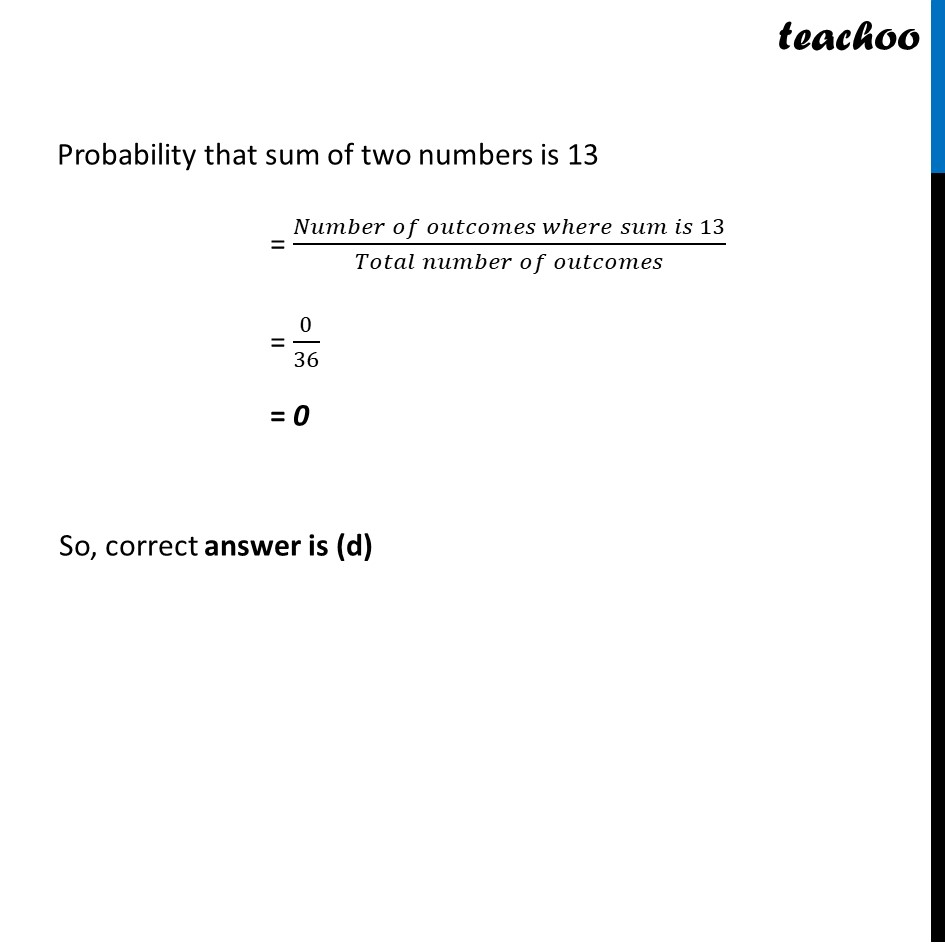## (a) 1                (b) 5/36              (c) 1/18                  (d) 0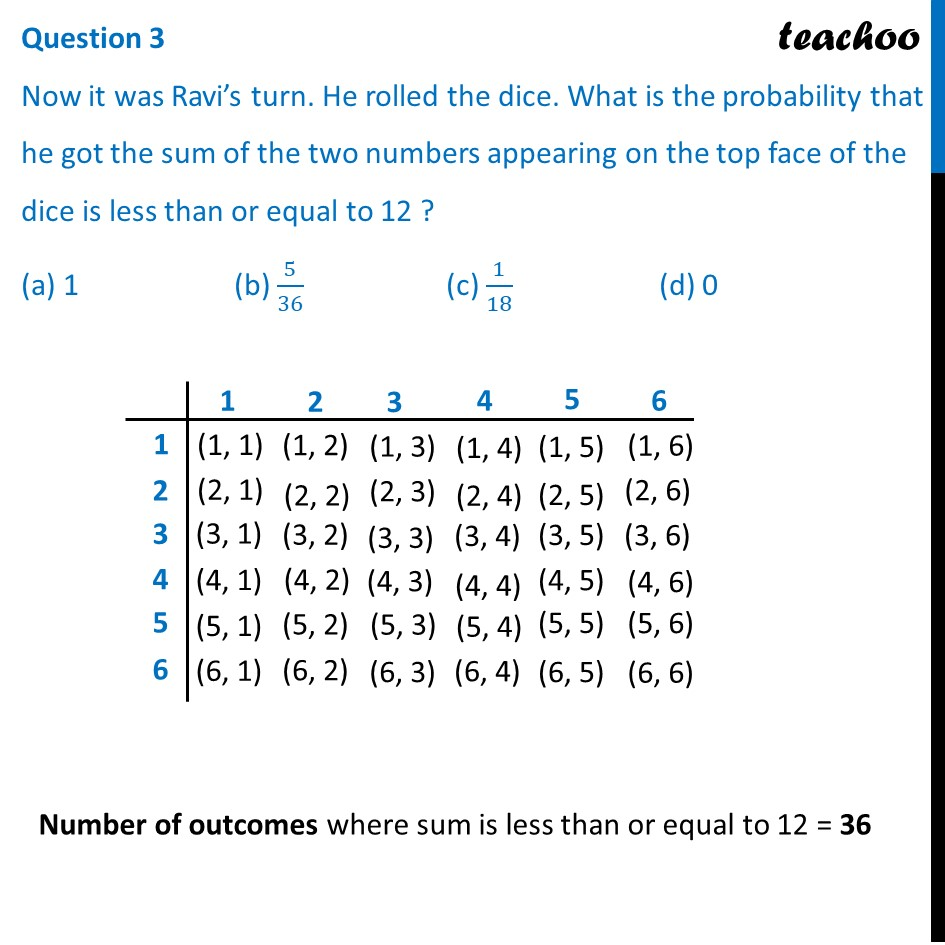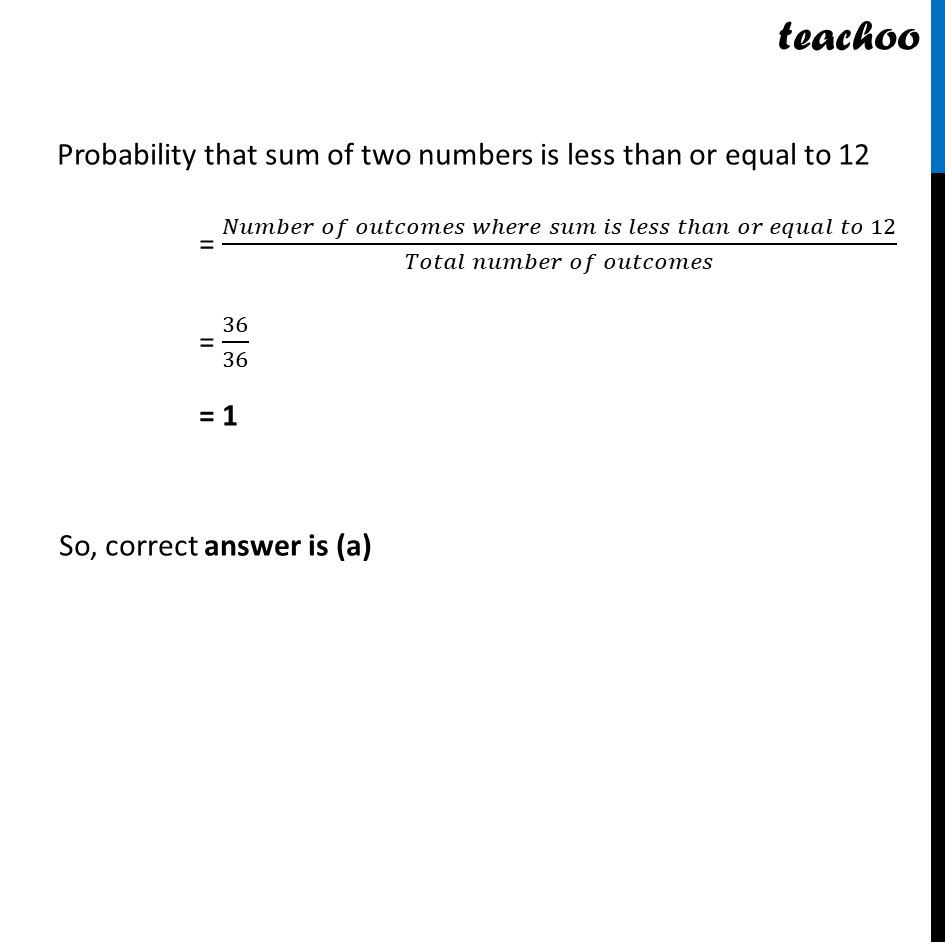## (a) 5/9              (b) 5/36               (c) 1/6                      (d) 0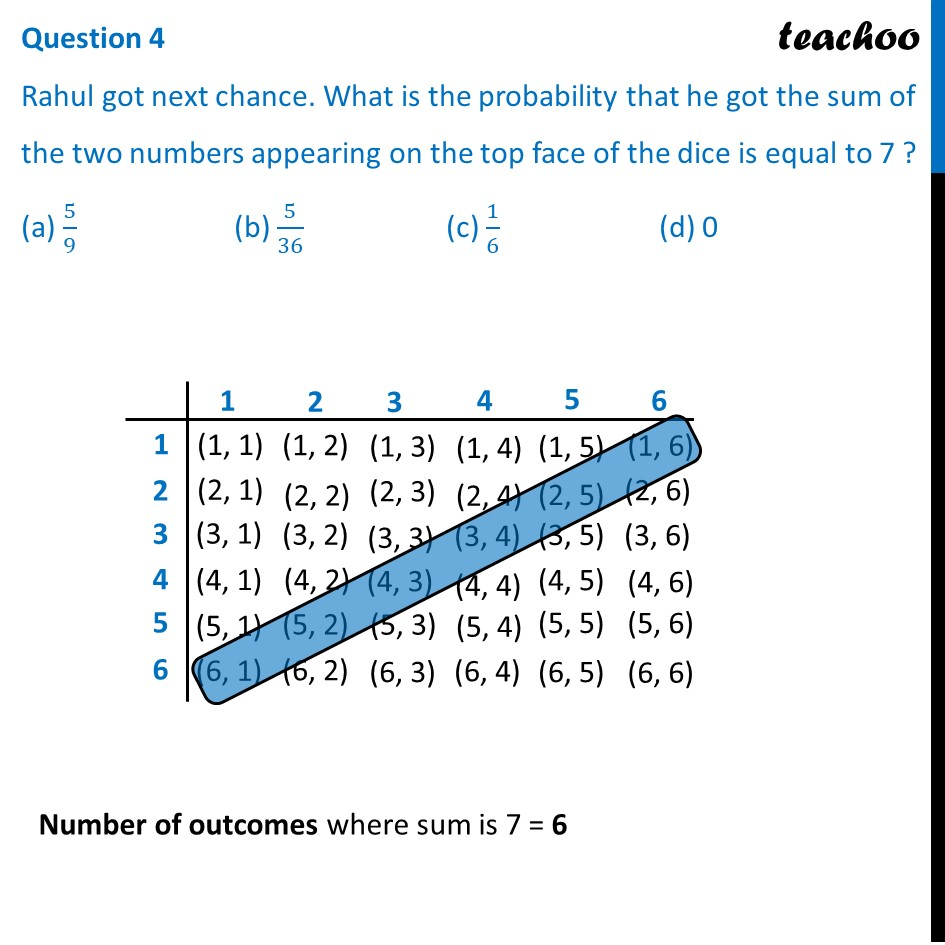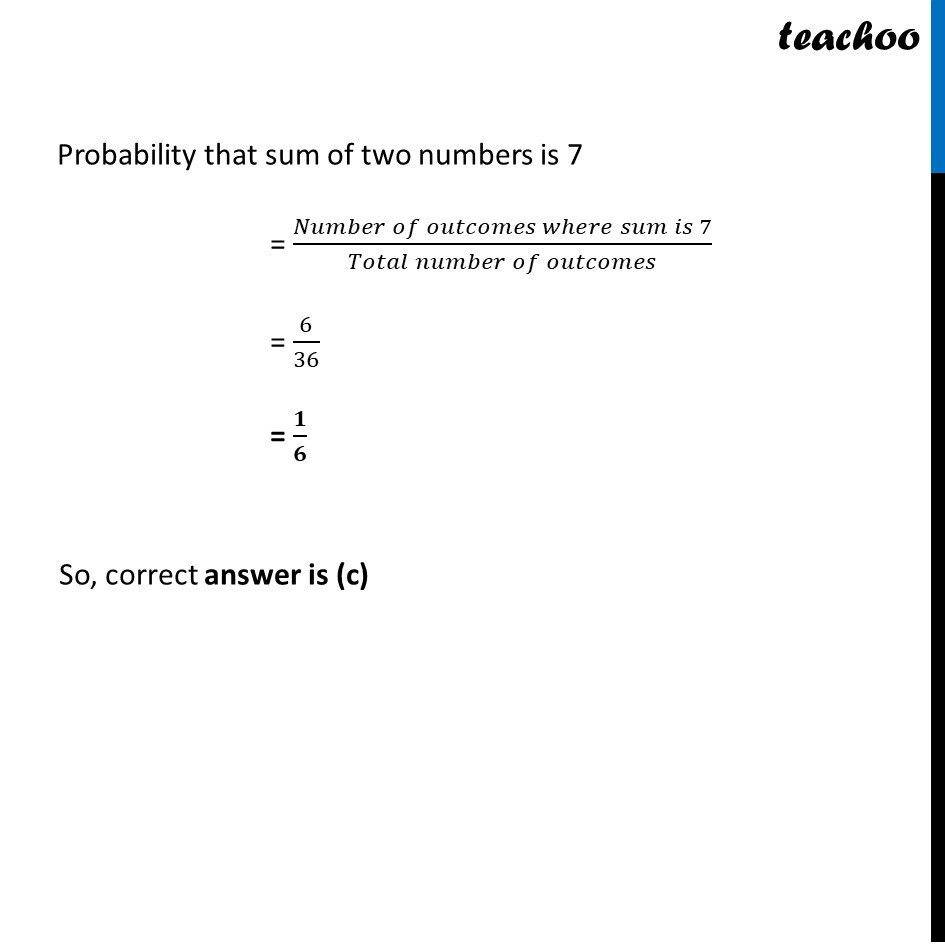## Question 5 Now it was Ravi’s turn. He rolled the dice. What is the probability that he got the sum of the two numbers appearing on the top face of the dice is greater than 8 ? (a) 1               (b) 5/36               (c) 1/18                        (d) 5/18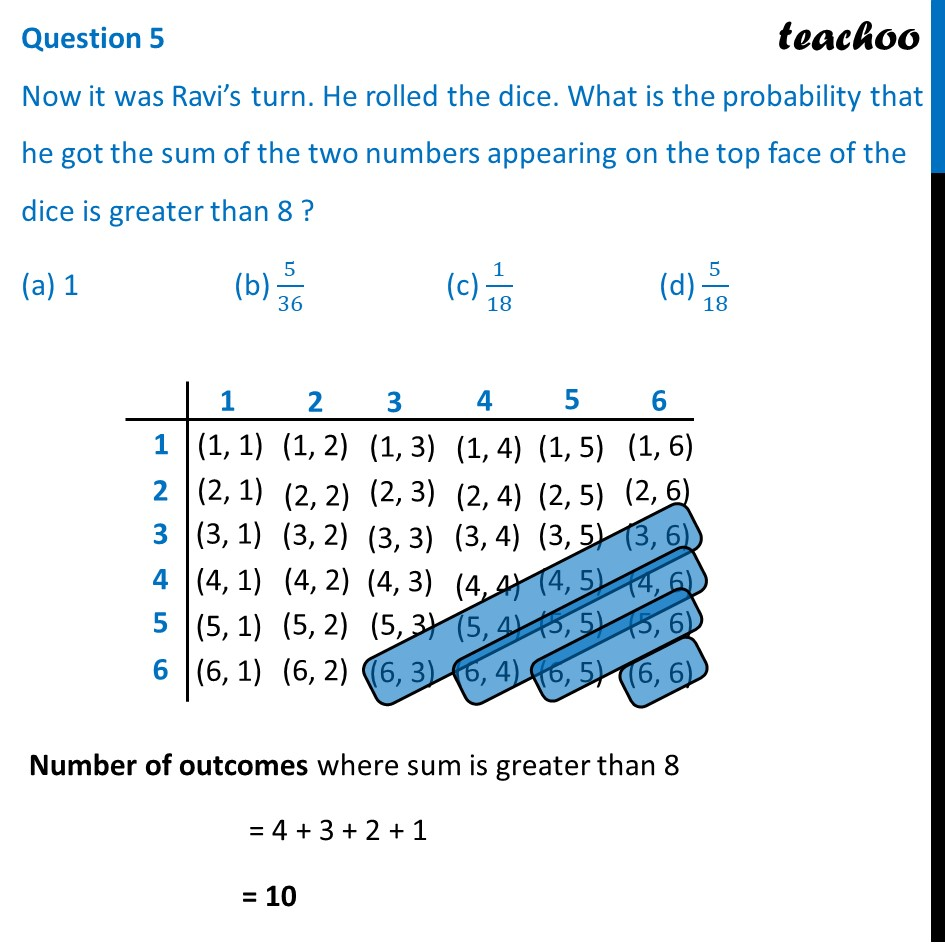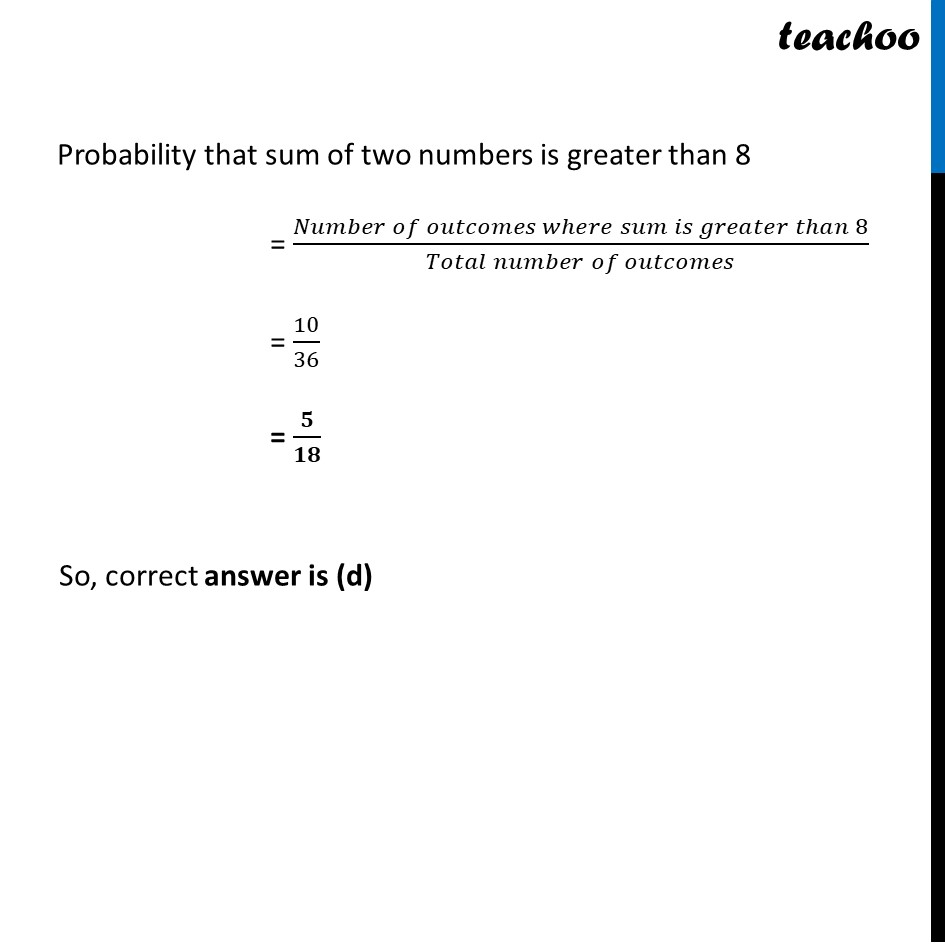1. Chapter 15 Class 10 Probability (Term 1)
2. Serial order wise
3. Case Based Questions (MCQ)

Transcript

Question Rahul and Ravi planned to play Business ( board game) in which they were supposed to use two diceFirst dice Second dice Question 1 Ravi got first chance to roll the dice. What is the probability that he got the sum of the two numbers appearing on the top face of the dice is 8? (a) 1/26 (b) 5/36 (c) 1/18 (d) 0Probability that sum of two numbers is 8 = (𝑁𝑢𝑚𝑏𝑒𝑟 𝑜𝑓 𝑜𝑢𝑡𝑐𝑜𝑚𝑒𝑠 𝑤ℎ𝑒𝑟𝑒 𝑠𝑢𝑚 𝑖𝑠 8)/(𝑇𝑜𝑡𝑎𝑙 𝑛𝑢𝑚𝑏𝑒𝑟 𝑜𝑓 𝑜𝑢𝑡𝑐𝑜𝑚𝑒𝑠) = 𝟓/𝟑𝟔 So, correct answer is (b) Question 2 Rahul got next chance. What is the probability that he got the sum of the two numbers appearing on the top face of the dice is 13? (a) 1 (b) 5/36 (c) 1/18 (d) 0Number of outcomes where sum is 13 = 0 Probability that sum of two numbers is 13 = (𝑁𝑢𝑚𝑏𝑒𝑟 𝑜𝑓 𝑜𝑢𝑡𝑐𝑜𝑚𝑒𝑠 𝑤ℎ𝑒𝑟𝑒 𝑠𝑢𝑚 𝑖𝑠 13)/(𝑇𝑜𝑡𝑎𝑙 𝑛𝑢𝑚𝑏𝑒𝑟 𝑜𝑓 𝑜𝑢𝑡𝑐𝑜𝑚𝑒𝑠) = 0/36 = 0 So, correct answer is (d) Question 3 Now it was Ravi’s turn. He rolled the dice. What is the probability that he got the sum of the two numbers appearing on the top face of the dice is less than or equal to 12 ? (a) 1 (b) 5/36 (c) 1/18 (d) 0Number of outcomes where sum is less than or equal to 12 = 36 Probability that sum of two numbers is less than or equal to 12 = (𝑁𝑢𝑚𝑏𝑒𝑟 𝑜𝑓 𝑜𝑢𝑡𝑐𝑜𝑚𝑒𝑠 𝑤ℎ𝑒𝑟𝑒 𝑠𝑢𝑚 𝑖𝑠 𝑙𝑒𝑠𝑠 𝑡ℎ𝑎𝑛 𝑜𝑟 𝑒𝑞𝑢𝑎𝑙 𝑡𝑜 12)/(𝑇𝑜𝑡𝑎𝑙 𝑛𝑢𝑚𝑏𝑒𝑟 𝑜𝑓 𝑜𝑢𝑡𝑐𝑜𝑚𝑒𝑠) = 36/36 = 1 So, correct answer is (a) Question 4 Rahul got next chance. What is the probability that he got the sum of the two numbers appearing on the top face of the dice is equal to 7 ? (a) 5/9 (b) 5/36 (c) 1/6 (d) 0Number of outcomes where sum is 7 = 6 Probability that sum of two numbers is 7 = (𝑁𝑢𝑚𝑏𝑒𝑟 𝑜𝑓 𝑜𝑢𝑡𝑐𝑜𝑚𝑒𝑠 𝑤ℎ𝑒𝑟𝑒 𝑠𝑢𝑚 𝑖𝑠 7)/(𝑇𝑜𝑡𝑎𝑙 𝑛𝑢𝑚𝑏𝑒𝑟 𝑜𝑓 𝑜𝑢𝑡𝑐𝑜𝑚𝑒𝑠) = 6/36 = 𝟏/𝟔 So, correct answer is (c) Question 5 Now it was Ravi’s turn. He rolled the dice. What is the probability that he got the sum of the two numbers appearing on the top face of the dice is greater than 8 ? (a) 1 (b) 5/36 (c) 1/18 (d) 5/18Number of outcomes where sum is greater than 8 = 4 + 3 + 2 + 1 = 10 Probability that sum of two numbers is greater than 8 = (𝑁𝑢𝑚𝑏𝑒𝑟 𝑜𝑓 𝑜𝑢𝑡𝑐𝑜𝑚𝑒𝑠 𝑤ℎ𝑒𝑟𝑒 𝑠𝑢𝑚 𝑖𝑠 𝑔𝑟𝑒𝑎𝑡𝑒𝑟 𝑡ℎ𝑎𝑛 8)/(𝑇𝑜𝑡𝑎𝑙 𝑛𝑢𝑚𝑏𝑒𝑟 𝑜𝑓 𝑜𝑢𝑡𝑐𝑜𝑚𝑒𝑠) = 10/36 = 𝟓/𝟏𝟖 So, correct answer is (d)

Case Based Questions (MCQ)

Chapter 15 Class 10 Probability (Term 1)
Serial order wise